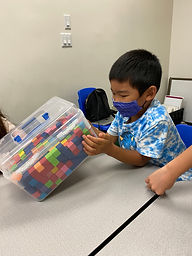## Ms. Brianne

### Target 1​

###### Lesson Type:

New

Number Operation

:

Computation

Estimate a given quantity based on place-value understandings.

###### 1:

Estimate quantities and use one-to-one correspondence to check the accuracy of the estimate.

###### 2:

Develop a strategy for estimating quantities (i.e., developing a unit of measurement for estimating – if this is what 10 units looks like this must be close to 40 units).

###### 3:

Make strategy adjustment based on the results of initial estimations.

###### 4:

Understand that rounding and estimation are a way to quickly check the accuracy of computations.

###### 5:

Understand that you can use estimation when an exact answer is not needed.

3rd

###### Vocabulary:

Estimation, Estimate

Activities:

• Students estimated the number of blocks in a small container. After everyone estimated the quantity, we counted the actual number of blocks to check for accuracy. Then they used that answer to help them adjust their new estimates for a larger container of blocks.
• Students were given pictures of objects and used a picture of the smaller set of the objects as a unit of measurement to help them make a more accurate estimate of the picture of the larger set of objects.
• Students added 2-digit numbers. They used estimation to check if their answer was reasonable. To estimate, they rounded each number to the nearest ten.### Home Exploration

###### Guiding Questions:## Absent Students:

### Target 2

:

###### 1:

Understand that a graph is a visual representation of data.

###### 2:

Become familiar with the vocabulary associated with graphs: unit, scale, title and labels.

###### 3:

Construct and read a simple line graph.

3rd

###### Vocabulary:

Data, Line Graph

Activities:

• Students were given a set of data of the number of pizzas sold each month and created a line graph by plotting the data points and connecting them. Then they used their line graph to answer questions about the data.
• Students used the data provided in a line graph about fishing to complete a data chart and answer questions.### Home Exploration

###### Guiding Questions:### Target 3

:

###### Vocabulary:

Activities:### Home Exploration